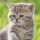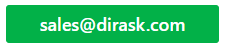Home
IT Knowledge
IT Career
Inspiration
Languages
EN

# JavaScript - how to calculate distance between two points in 3D space?

5 points
Created by:Kate_C
19800

Quick solution:

``````// P1 = (x1, y1, z1); P2 = (x2, y2, z2)

var a = x2 - x1;
var b = y2 - y1;
var c = z2 - z1;

var distance = Math.sqrt(a * a + b * b + c * c);``````

## 1. Overview

Distance calculation between two points is possible with midified Pythagorean equation. In this article, example how to calculate the distance is presented.

## 2. Mathematical theorem for coordinate system

Distance calculation for points requires to start with transformation of Pythagorean equation to point version.

``````a^2 + b^2 + c^2 = d^2 => d = sqrt(a^2 + b^2 + c^2)

P1 = (x1, y1, z1); P2 = (x2, y2, z2)

a = |x2 - x1|
b = |y2 - y1|
c = |z2 - z1|

d = sqrt(|x2 - x1|^2 + |y2 - y1|^2 + |z2 - z1|^2)``````

what can be transformed to:

``````d = sqrt((x2 - x1)^2 + (y2 - y1)^2 + (z2 - z1)^2)

Distance between P1 and P2 is equal to d.``````

Note: absolute values can be avoided because of squares inside Pythagorean equation - squares remove minuses.

Example calculations:

``````P1 = (7, 2, 3); P2 = (3, 5, 8)

a = |x2 - x1| = |3 - 7| = 4
b = |y2 - y1| = |5 - 2| = 3
c = |z2 - z1| = |8 - 3| = 5

d = sqrt(4^2 + 3^2 + 5^2) = sqrt(16 + 9 + 25) = sqrt(50)
d = 7.071067811865475``````

## 3. JavaScript custom distance function example

``````// ONLINE-RUNNER:browser;

function calculateDistance(p1, p2) {
var a = p2.x - p1.x;
var b = p2.y - p1.y;
var c = p2.z - p1.z;

return Math.sqrt(a * a + b * b + c * c);
}

// Example:

var p1 = {x: 7, y: 2, z: 3};
var p2 = {x: 3, y: 5, z: 8};

var distance = calculateDistance(p1, p2);

console.log(distance);

``````

## 4. Math.hypot method example

Note: `Math.hypot` has been introduced in ECMAScript 2015.

``````// ONLINE-RUNNER:browser;

function calculateDistance(p1, p2) {
var a = p2.x - p1.x;
var b = p2.y - p1.y;
var c = p2.z - p1.z;

return Math.hypot(a, b, c);
}

// Example:

var p1 = {x: 7, y: 2, z: 3};
var p2 = {x: 3, y: 5, z: 8};

var distance = calculateDistance(p1, p2);

console.log(distance);``````

1. JavaScript - how to calculate distance between two points with Pythagorean equation?